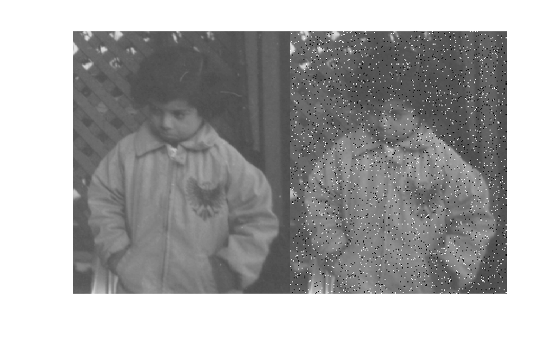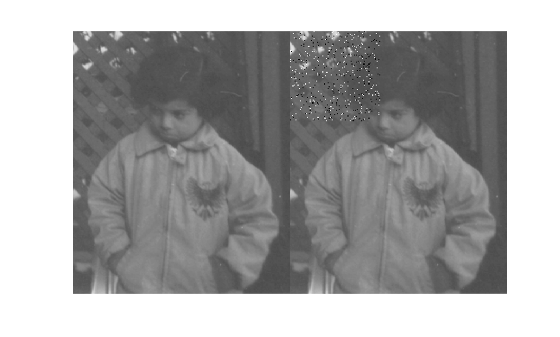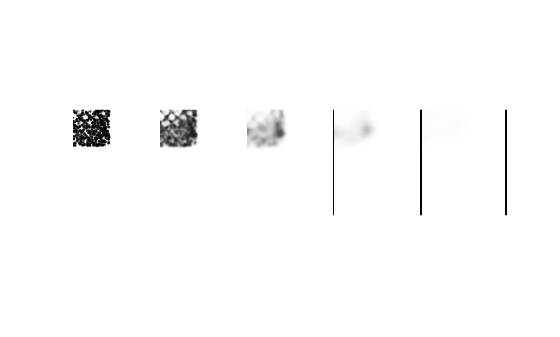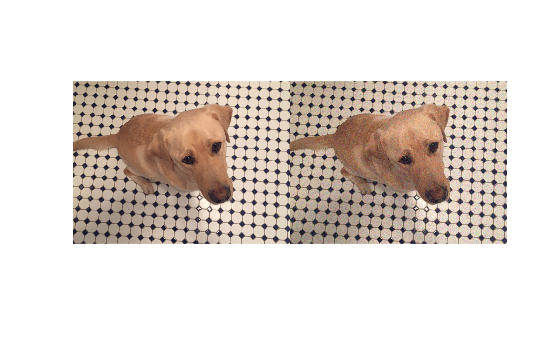# multissim

Multiscale structural similarity (MS-SSIM) index for image quality

Since R2020a

## Syntax

``score = multissim(I,Iref)``
``score = multissim(I,Iref,Name,Value)``
``[score,qualityMaps] = multissim(___)``

## Description

example

````score = multissim(I,Iref)` calculates the multi-scale structural similarity (MS-SSIM) index, `score`, for image `I`, using `Iref` as the reference image. A value closer to 1 indicates better image quality and a value closer to 0 indicates poorer quality.MS-SSIM is only defined for grayscale images. For inputs with more than two dimensions, `multissim` treats each element of higher dimensions as a separate 2-D grayscale image.```

example

````score = multissim(I,Iref,Name,Value)` controls aspects of the computation using one or more name-value arguments. For example, specify the number of scales using the `'NumScales'` argument.```

example

````[score,qualityMaps] = multissim(___)` also returns the local MS-SSIM index value for each pixel in each scaled version of `I`. The `qualitymap` output is a cell array containing maps for each of the scaled versions of `I`. Each quality map is the same size as the corresponding scaled version of `I`.```

## Examples

collapse all

Load an image into the workspace.

`Iref = imread('pout.tif');`

Create a noisy version of the image for comparison purposes.

`I = imnoise(Iref,'salt & pepper',0.05);`

Display the original image and noisy image.

```figure; montage({Iref,I}); ```Calculate the MS-SSIM index that measures the quality of the input image compared to the reference image.

`score = multissim(I,Iref)`
```score = single 0.6732 ```

Load an image into the workspace.

```Iref = imread('pout.tif'); I = Iref;```

Add noise to a localized part of the image.

`I(1:100,1:100) = imnoise(Iref(1:100,1:100),'salt & pepper',0.05);`

Display the original image and the noisy image.

```figure; montage({Iref,I});```Calculate the local MS-SSIM index maps for the noisy image, `qualitymaps`, using the original image as the reference. The return value, `qualitymaps`, is a cell array containing a quality map for each of the scaled versions of the image. Each map is the same size as the corresponding scaled version of the image.

```[~, qualitymaps] = multissim(I,Iref); figure montage(qualitymaps,'Size',[1 5])```Load an image into the workspace.

`Iref = imread('pout.tif');`

Create a noisy version of the image for comparison purposes.

`I = imnoise(Iref,'salt & pepper',0.05);`

Display the original image and the noisy version of the image.

```figure; montage({Iref,I});```Calculate the MS-SSIM index for the noisy image, using the original image as the reference. Specify how much to weigh the local MS-SSIM index calculations for each scaled image, using the `'ScaleWeights'` argument. The example uses the weight values defined in the article by Wang, Simoncelli, and Bovik.

`score = multissim(I,Iref,'ScaleWeights',[0.0448,0.2856,0.3001,0.2363,0.1333])`
```score = single 0.6773 ```

Read a color image into the workspace.

`RGB = imread("kobi.png");`

Create a version of the image with added salt and pepper noise.

`RGBNoisy = imnoise(RGB,"salt & pepper");`

Display the two images in a montage.

`montage({RGB,RGBNoisy})`Calculate the MS-SSIM of each color channel of the noisy image.

```score = multissim(RGBNoisy,RGB); score = squeeze(score)```
```score = 3x1 single column vector 0.7084 0.7135 0.7066 ```

Read a color image into the workspace.

```ref = imread("strawberries.jpg"); ref = im2single(ref);```

Simulate a batch of six images by replicating the image along the fourth dimension.

`refBatch = repmat(ref,[1 1 1 6]);`

Create a copy of the batch of images, adding salt and pepper noise.

`noisyBatch = imnoise(refBatch,"salt & pepper");`

Create a formatted `dlarray` object for the original and noisy batch of images. The format is "SSCB" for spatial-spatial-channel-batch.

```dlrefBatch = dlarray(refBatch,"SSCB"); dlnoisyBatch = dlarray(noisyBatch,"SSCB");```

Calculate the MS-SSIM score of the noisy data with respect to the original data.

`scores = multissim(dlnoisyBatch,dlrefBatch);`

Remove the singleton dimensions corresponding to the spatial dimensions and display the scores. Each element is the MS-SSIM score for one color channel of one image of the batch.

`squeeze(scores)`
```ans = 3(C) x 6(B) single dlarray 0.8334 0.8335 0.8348 0.8335 0.8340 0.8349 0.8325 0.8316 0.8309 0.8310 0.8317 0.8326 0.8140 0.8123 0.8166 0.8129 0.8136 0.8123 ```

## Input Arguments

collapse all

Input image, specified as a numeric array of any dimension or a `dlarray` (Deep Learning Toolbox) object. Formatted `dlarray` objects cannot include more than one channel label, more than one batch label, and more than two spatial labels.

Data Types: `single` | `double` | `int16` | `uint8` | `uint16`

Reference image, specified as a numeric array of any dimension or a `dlarray` (Deep Learning Toolbox) object. Formatted `dlarray` objects cannot include more than one channel label, more than one batch label, and more than two spatial labels.

Data Types: `single` | `double` | `int16` | `uint8` | `uint16`

### Name-Value Arguments

Specify optional pairs of arguments as `Name1=Value1,...,NameN=ValueN`, where `Name` is the argument name and `Value` is the corresponding value. Name-value arguments must appear after other arguments, but the order of the pairs does not matter.

Before R2021a, use commas to separate each name and value, and enclose `Name` in quotes.

Example: `score = multissim(I,Iref,"NumScales",3);`

Number of scales used to calculate the MS-SSIM index, specified as a positive integer. Setting `NumScales` to `1` is equivalent to using the `ssim` function with its `Exponents` name-value argument set to ```[1 1 1]```. The size of the input image limits the number of scales. The `multissim` function scales the image (`NumScales` - 1) times, downsampling the image by a factor of 2 with each scaling.

Data Types: `single` | `double` | `int8` | `int16` | `int32` | `int64` | `uint8` | `uint16` | `uint32` | `uint64`

Relative values across the scales, specified as a vector of positive elements. The length of the vector is equal to the number of scales, because each element corresponds to one of the scaled versions of the original image. The `multissim` function normalizes the values to 1. By default, the scale weights equal `fspecial``("gaussian",[1,numScales],1)`. The `multissim` function uses a Gaussian distribution as the default because the human visual sensitivity peaks at middle frequencies and decreases in both directions. For an example of setting `ScaleWeights`, see Calculate MS-SSIM Specifying Scale Weights.

Data Types: `single` | `double` | `int8` | `int16` | `int32` | `int64` | `uint8` | `uint16` | `uint32` | `uint64`

Standard deviation of the isotropic Gaussian function, specified as a positive number. This value specifies the weighting of the neighborhood pixels around a pixel for estimating local statistics. The `multissim` function uses weighting to avoid blocking artifacts when estimating local statistics.

Data Types: `single` | `double` | `int8` | `int16` | `int32` | `int64` | `uint8` | `uint16` | `uint32` | `uint64`

Dynamic range of the input image, specified as a positive number. The default value of `DynamicRange` depends on the data type of image `I`, and is calculated as `diff(getrangefromclass(I))`. For example, the default dynamic range is `255` for images of data type `uint8`, and the default is `1` for images of data type `double` or `single` with pixel values in the range [0, 1].

Data Types: `single` | `double` | `int8` | `int16` | `int32` | `uint8` | `uint16` | `uint32`

## Output Arguments

collapse all

MS-SSIM index for image quality, returned as a numeric scalar, numeric array, or `dlarray` (Deep Learning Toolbox) object as indicated in the table. The value of `score` is typically in the range [0, 1]. The value 1 indicates the highest quality and occurs when `I` and `Iref` are equivalent. Smaller values correspond to poorer quality. For some combinations of inputs and name-value pair arguments, `score` can be negative.

Input Image TypeMS-SSIM Value
2-D numeric matricesNumeric scalar with a single MS-SSIM measurement.
2-D `dlarray` objects1-by-1 `dlarray` object with a single MS-SSIM measurement.
N-D numeric arrays with N>2Numeric array of the same dimensionality as the input images. The first two dimensions of `score` are singleton dimensions. There is one MS-SSIM measurement for each element along the higher dimensions.

Unformatted N-D `dlarray` objects with N>2

`dlarray` object of the same dimensionality as the input images. The first two dimensions of `score` are singleton dimensions. There is one MS-SSIM measurement for each element along the higher dimensions.

Formatted N-D `dlarray` objects with N>2

`dlarray` object of the same dimensionality as the input images. The spatial dimensions of `score` are singleton dimensions. There is one MS-SSIM measurement for each element along any channel or batch dimension.

Local MS-SSIM index values for each pixel in each scaled version, returned as a cell array of numeric arrays or a cell array of `dlarray` (Deep Learning Toolbox) objects. The size of the cell array is 1-by-`NumScales`. Each element in `qualityMaps` indicates the quality of the corresponding pixel at the corresponding scale factor. The format of each element uses the formatting of the `scores` argument, based on the format of the input images.

## Algorithms

The structural similarity (SSIM) index measures perceived quality by quantifying the SSIM between an image and a reference image (see `ssim`). The `multissim` function calculates the MS-SSIM index by combining the SSIM index of several versions of the image at various scales. The MS-SSIM index can be more robust when compared to the SSIM index with regard to variations in viewing conditions.

The `multissim` function uses double-precision arithmetic for input images of class `double`. All other types of input images use single-precision arithmetic.

 Wang, Z., Simoncelli, E.P., Bovik, A.C. Multiscale Structural Similarity for Image Quality Assessment. In: The Thirty-Seventh Asilomar Conference on Signals, Systems & Computers, 2003, 1398–1402. Pacific Grove, CA, USA: IEEE, 2003. https://doi.org/10.1109/ACSSC.2003.1292216.